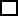Create a new printableFractions
Math Worksheets

Sample - Click above to make a new math worksheet (PDF).
 Name _____________________________Date ___________________
Number Theory
Classify each number as prime or composite.
 1 40PrimeComposite
 2 66PrimeComposite
 3 * This is a pre-made sheet.Use the link at the top of the page for a printable page.
 4 30PrimeComposite
 5 5PrimeComposite
 6 57PrimeComposite
 7 82PrimeComposite
 8 29PrimeComposite

Complete each divisibility table. Write yes if the number is divisible by the given number. Write no if it is not divisible by the given number.
9.         53
 by 2 ________ by 3 ________ by 8 ________ by 9 ________ by 10 ________
10.         144
 by 4 ________ by 5 ________ by 6 ________ by 7 ________ by 8 ________
11.         9,511
 by 3 ________ by 7 ________ by 8 ________ by 9 ________ by 12 ________
12.         2,317
 by 3 ________ by 5 ________ by 7 ________ by 10 ________ by 13 ________

Use the clue to fill in the missing digit.
 13 The number04 is divisible by 11.
 14 The number 57 is divisible by 17.
 15 The number98 is divisible by 7.
 16 The number620 is divisible by 5.
 17 The number 191 is divisible by 3.
 18 The number 11is divisible by 19.
 19 The number680 is divisible by 6.
 20 The number92 is divisible by 16.

List all of the factors of each number.
 21 47
 22 35
 23 14
 24 39
 25 61
 26 23
 27 36
 28 38
 29 80
 30 67
 31 98
 32 68

 Key #2
 aKey #2
Find the prime factorization of each number.
 33 80
 34 32
 35 33
 36 85
 37 55
 38 22
 39 21
 40 70
 41 67
 42 92
 43 95
 44 77

Find the greatest common factor of each set of numbers.
 45 48 and 36
 46 85 and 40
 47 3 and 76
 48 96 and 60
 49 18, 36, and 27
 50 96, 88, and 92
 51 63 and 54
 52 44 and 59
 53 24 and 12

Find the least common multiple.
 54 3 and 7
 55 3 and 5
 56 16 and 22
 57 6, 14, and 15
 58 8 and 20
 59 10 and 12
 60 4 and 10
 61 2, 8, and 13
 62 3, 9, and 18

Complete.
 63 Identify two numbers whose GCF is 24 and whose LCM is 144. Describe how you found the number.
 64 Numbers that end in zero are considered even because they are definitely divisible by two. However, the number 0 is neither even nor odd. Think about this, and give an explanation of why this must be true.